Journal of Multimedia Information System
Korea Multimedia Society
Section A

# Efficient Superpixel Generation Method Based on Image Complexity

Sanghyun Park1,*
1Department of Multimedia Engineering, Sunchon National University, Jeonnam, Korea, shark@scnu.ac.kr
*Corresponding Author: Sanghyun Park, 255 Jungang-ro, Sunchon, Jeonnam, 57922 Korea, +82-61-750-3833, shark@scnu.ac.kr.

© Copyright 2020 Korea Multimedia Society. This is an Open-Access article distributed under the terms of the Creative Commons Attribution Non-Commercial License (http://creativecommons.org/licenses/by-nc/4.0/) which permits unrestricted non-commercial use, distribution, and reproduction in any medium, provided the original work is properly cited.

Received: Sep 04, 2020; Revised: Sep 04, 2020; Accepted: Sep 21, 2020

Published Online: Sep 30, 2020

## Abstract

Superpixel methods are widely used in the preprocessing stage as a method to reduce computational complexity by simplifying images while maintaining the characteristics of the images in the computer vision applications. It is common to generate superpixels of similar size and shape based on the pixel values rather than considering the characteristics of the image. In this paper, we propose a method to control the sizes and shapes of generated superpixels, considering the contents of an image. The proposed method consists of two steps. The first step is to over-segment an image so that the boundary information of the image is well preserved. In the second step, generated superpixels are merged based on similarity to produce the target number of superpixels, where the shapes of superpixels are controlled by limiting the maximum size and the proposed roundness metric. Experimental results show that the proposed method preserves the boundaries of the objects in an image more accurately than the existing method.

Keywords: Agglomerative clustering; graph algorithm; image segmentation; superpixels

## I. INTRODUCTION

Image segmentation is one of the traditional research areas of image processing. The purpose of image segmentation is usually to recognize the objects in an image . Image segmentation is also used as a method to reduce the computational amount when processing a lot of images. Since an image consists of many pixels, it is necessary to reduce the amount of computation when processing many images. For this purpose, an image is divided into groups of similar pixels, and each pixel group is processed like one pixel. A group of similar pixels is called a superpixel , .

A good superpixel method reduces the resolution of an image but maintains the boundaries of the objects in the image. Therefore, we can reduce the overfitting of images and unnecessary noises in image processing applications using superpixels. Superpixel technique is commonly used as a preprocessing stage in computer vision applications such as lane detection algorithms.

Various methods for generating superpixels have been proposed, such as the mean shift methods  and the graph-based methods . There are three criteria for evaluating good superpixels -. First, one superpixel must have similar color characteristics, and the next requirement is to preserve the boundaries of objects in the image well. The last requirement is similar sizes and shapes of superpixels.

The criteria required in superpixels have trade-off relationships with each other, so it is difficult to improve all characteristics at the same time. Therefore, it is proper to generate superpixels focusing on specific purposes according to the requirements of the application. For example, for the applications that track objects, superpixels of similar shapes are more advantageous in finding objects than superpixels that preserve the exact boundary of objects .

Since superpixel methods are used in the preprocessing stage of the computer vision applications, many existing algorithms keep similar shapes of superpixels. When a target number of superpixels is given, each superpixel is generated to be similar in size to the average size of superpixels, which is the value of dividing the total number of pixels by the target number of superpixels. However, the complexities of the areas in an image are different. In order to preserve the boundaries of the objects, a complex area requires many superpixels, while a simple area requires a small number of superpixels. In this paper, we propose a superpixel generation method that preserves the boundaries of objects well by reflecting the complexity of the areas in an image.

## II. RELATED WORK

2.1. Superpixel-based computer vision algorihtms

There are a lot of studies on applying artificial intelligence technology to image processing. Since an image has a large amount of data, a lot of research is also being conducted to reduce the amount of computation by using superpixels. Image processing algorithms using superpixels can be divided into the methods where the boundary information of the objects is important, and the methods where the boundary information is not critical. When detecting whether a fight is taking place in an image, it is important to detect movement changes of objects . When recognizing signals expressed by hand, it is important to recognize the geometric shape of the fingers . In these cases, it is advantageous to generate superpixels of similar shapes.

On the other hand, when superpixels are used in applications that search for a certain content in images, boundary information becomes important , . In applications that recognize human facial expressions, it is important to accurately detect boundaries in order to capture subtle changes in facial expressions . In these cases, superpixels that are irregular but preserve the boundaries is advantageous. Especially in the case of medical images, it is important to preserve the boundaries well. In applications that automatically detect tumors in MRI images or detect liver regions in CT images, preserving boundaries well affects the performance , .

Currently, many applications use the SLIC (Simple Linear Iterative Clustering) method to create superpixels . SLIC algorithm performs better in adhering to image boundaries, speed, and memory efficiency compared with existing superpixel algorithms. However, in the case of images containing different elements with complex patterns such as medical images, the SLIC method cannot accurately detect the boundaries. It remains challenging to have the boundary properly covered by superpixels especially for the superpixels at the border of the target area such as liver region in CT images .

2.2. Simple Linear Iterative Clustering

The proposed method consists of two steps. In the first step, a sufficient number of superpixels are generated to preserve the boundaries of objects well using the existing method. In the second step, adjacent similar superpixels are merged to create the final result. In this paper, we use the SLIC method for the first step. Thus, before describing the proposed method, we review the SLIC method .

The SLIC algorithm first changes the color model from RGB to CIELAB for the input image. The next step is to establish k initial clustering centers, where k is the target number of superpixels. The initial centers are set at a regular interval. When the number of pixels of the image is A, the interval between the initial centers is determined by Eq. (1).

(1)

If the centers of the clusters are determined, similarity metrics between a pixel and the cluster centers in the region of 2S × 2S are calculated. That is, a pixel compares similarity with the initial centers, which are in the region of 2S × 2S, and then it is clustered into the most similar cluster center. The similarity is measured by the distance, and the closer the distance, the higher the similarity. The distance between a pixel and a cluster center is determined by considering not only the color component but also the location. The feature vector of pixel i is composed of the color information (li, ai, and bi) and position information (xi and yi). The feature vector of the kth cluster Ck is also composed of the color information (lCk, aCk, and bCk) and the position information (xCk and yCk) as

(2)

A distance metric between pixel i and cluster Ck is defined as

(3)
(4)
(5)

where λ = r/S, and r is the weight used to combine Dc, which is the distance measured using color information, and Ds, which is the distance measured using location information. In the SLIC algorithm, each pixel compares the distances for cluster centers in the 2S × 2S area around the pixel to determine its cluster. Therefore, the SLIC algorithm has a constant number of distance calculations regardless of the number of clusters. Also, since each pixel calculates its distance only to its adjacent cluster centers, the SLIC algorithm can quickly generate superpixels.

Fig. 1 shows the results of generating superpixels by applying the SLIC algorithm to Bee image. Bee image consists of simple backgrounds and a bee and flowers that show intricate patterns. As shown in the figure, the SLIC algorithm segments the simple background into superpixels of relatively constant size. It can also be seen that the boundaries of the bee area and flower area having a complex pattern are not well preserved. Depending on the application, it can be more efficient to adjust the size of the superpixel by reflecting the complexity of the area in the image rather than to divide the image into superpixels of similar sizes.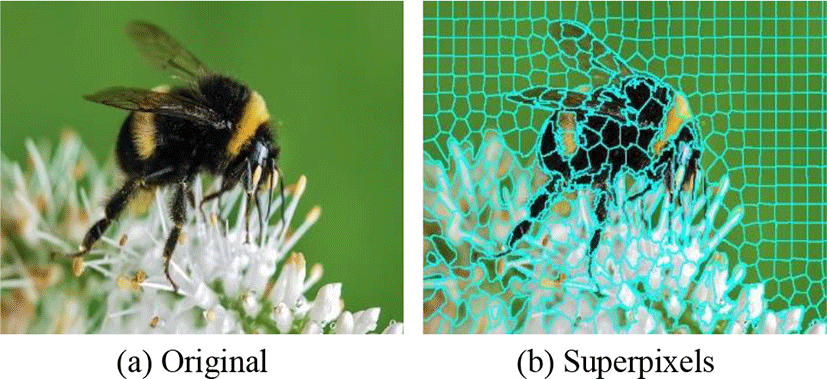Fig. 1. Bee image and its superpixel image segmented using SLIC algorithm.

## III. PROPOSED SUPERPIXEL GENERATION METHOD

The proposed method generates the target number of superpixels by merging the over-segmented superpixels, which are generated using the SLIC algorithm. In the proposed scheme, the feature vector of each superpixel defined by Eq. (2) is modified, as shown in Eq. (6).

(6)

where nCk denotes the number of pixels constituting the superpixel, and pCk represents the index of the parent superpixel. pCk is initialized to Ck value, which means pCk points to iteself. In agglomerative clustering, if pCk refers to itself, Ck is the top superpixel of the cluster. When superpixel Ci and superpixel Cj merge, if the index i is smaller than j, the superpixel Ci becomes the parent, and superpixel Cj is merged into superpixel Ci. That is, superpixel Cj becomes a child of superpixel Ci, so pCj is updated to Ci.

The proposed method merges superpixels using the graph-based agglomerative clustering method . Therefore, the first step to merging is to create a graph of over-segmented superpixels. A graph of superpixels G consists of the node set V and the edge set E. Each node corresponds to each over-segmented superpixel. Thus, if the number of over-segmented superpixels is N, the number of nodes is N. An edge is defined between adjacent two superpixels, so the number of edges M is determined by the connection state of the entire superpixels.

(7)
(8)
(9)

where ei is the ith edge. Each edge is defined by three elements, as shown in Eq. (10). The first two elements of an edge are superpixel Ci and superpixel Cj that constitute the edge. The third element of Eq. (10) is the weight w indicating the distance between the two superpixels. The edge distance De between two superpixels is defined as the norm of the color component difference vector of two superpixels as Eq. (12).

${e}_{k}=\left({C}_{i},{C}_{j},{w}_{k}\right),$
(10)
${w}_{k}={D}_{e}\left({C}_{i},{C}_{j}\right),$
(11)
${D}_{e}\left({C}_{i},{C}_{j}\right)=\sqrt{{\left({l}_{{C}_{i}}-{l}_{{C}_{j}}\right)}^{2}+{\left({a}_{{C}_{i}}-{a}_{{C}_{j}}\right)}^{2}+{\left({b}_{{C}_{i}}-{b}_{{C}_{j}}\right)}^{2}}.$
(12)

Once the graph is completed, the edges are sorted based on w. In the sorted edge set E, edges are selected in order, and the superpixels comprising the selected edge are merged. If superpixel Ci and superpixel Cj are merged, and i is less than j, superpixel Cj becomes a child of superpixel Ci. Thus, the feature vector fCi is updated with the information of the merged superpixel. In the case of Cj, only pCk is updated to Ci.

The proposed method limits the size of the merged superpixel. That is, two superpixels are merged only when the number of pixels constituting the two superpixels is less than the maximum size. The maximum size MS is controlled by the parameter α indicating a multiple for the average size of superpixels as Eq. (13).

$MS=\text{α}\cdot \text{A}/{N}^{*},$
(13)

where N* is the target number of superpixels. Thus, A/N* is the average number of pixels of final superpixels.

In addition, the proposed method controls the shapes of superpixels. When we limit the maximum size of each superpixel, the shape of each superpixel can be very irregular. We assume that the ideal shape of a superpixel is a circle. When the number of pixels constituting a superpixel is AC, and the superpixel has the ideal circle form, the radius r of the superpixel is approximated as Eq. (14).

$r=\sqrt{{A}_{C}/\pi }$
(14)

Fig. 2 shows an example of a superpixel created by merging several superpixels. In the figure, a square means a superpixel created by the SLIC method. The actual superpixels vary in form, but they are assumed in the same form to explain the concept of roundness metric easily. Let the number of pixels of the merged superpixel be AC. The circle in Fig. 2 is a circle with the radius r centered on the average coordinate of all pixels. Let Ao denote the number of pixels that make up the superpixels whose centers are located outside this circle. The roundness of the merged superpixel can then be defined as Eq. (15).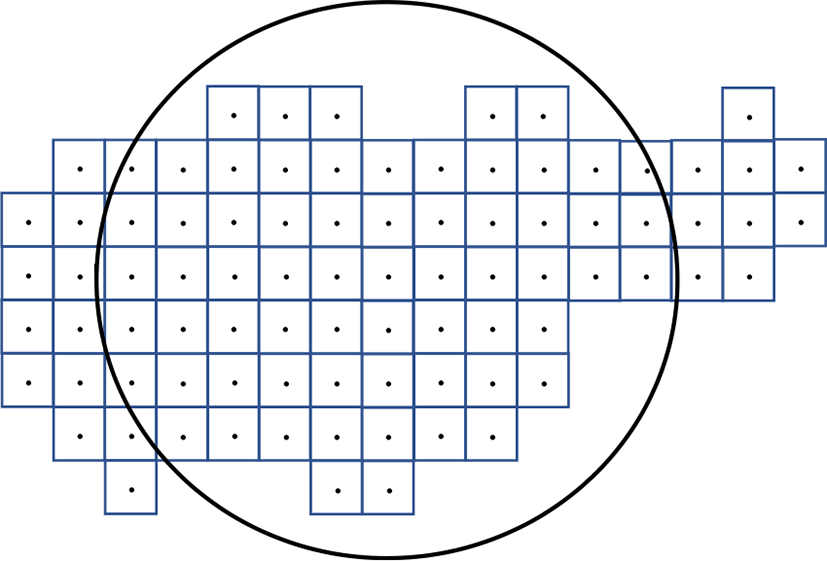Fig. 2. Roundness measurement example.
$R=1-\frac{{A}_{o}}{{A}_{C}}.$
(15)

As the shape of a superpixel is closer to the circle, the roundness metric R is closer to 1. When two superpixels are candidates to merge, the roundness value R is calculated for two superpixels, and they are merged only when the roundness value is greater than the threshold ThR.

Algorithm 1 describes the proposed superpixel generation method. The algorithm basically repeats the process of merging superpixels until the number of superpixels reaches N*. From the sorted E, edge et is extracted in order. Merging is made through the parent superpixel, so we look for the parent superpixels of the two superpixels that make up the edge. If parent superpixels are the same, it means that two superpixels have already been merged. If parent superpixels are different, the two superpixels can be merged. Before two superpixels merging, it should be confirmed that two superpixels satisfy the size constraint and the roundness constraint.

Algorithm 1: Algorithm for the proposed superpixel generation method

Input: G(V, E), N, N*, MS, and ThR

While N > N*

Take out et = (C1t, C2t, wt) from the sorted E

C1 = find_parent(C1t)

C2 = find_parent(C2t)

if(C1 = C2) continue

if(nC1 + nC2 > MS) continue

if(Roundness(C1, C2) < ThR) continue

Merge(C1, C2)

N = N − 1

end

Output: Updated G(V, E)

## IV. EXPERIMENTAL RESULTS

In order to verify the performance of the proposed method, we compare the results of the proposed method with the results of the SLIC algorithm. First, we generate superpixels by applying the SLIC algorithm to Bee image, which is used in the algorithm description. Fig. 3 shows the result of superpixel segmentation with the target number of 300. The other parameters of the SLIC algorithm are set to the default values.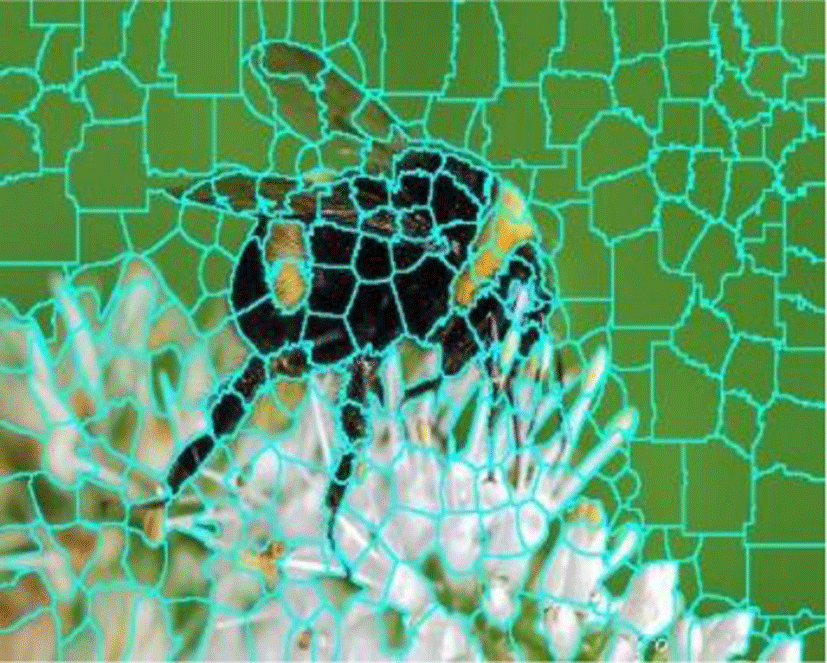Fig. 3. Superpixel image generated by the SLIC method.

The proposed method can control the maximum size of a superpixel with parameter α. When the target number of superpixels is given, the average size of superpixels is determined. Then the maximum size of a superpixel is calculated as α times the average size. Fig. 4 shows the results of generating 300 superpixels with various α values. As the α value increases, the sizes of superpixels become more diverse. We can also see that the sizes of superpixels are determined according to the complexity of the area in the image.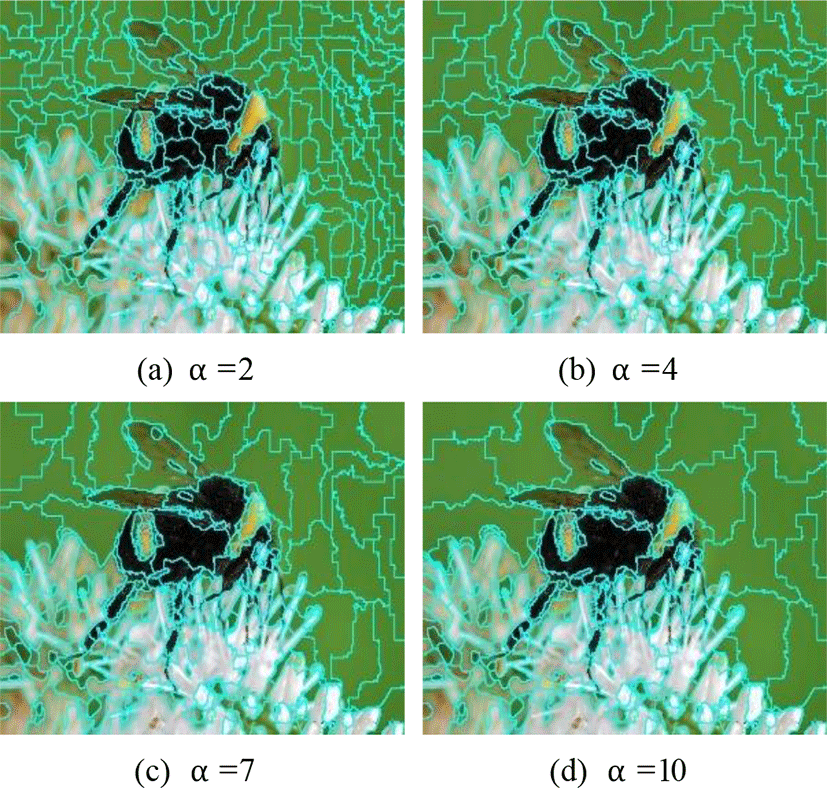Fig. 4. Superpixel images with various maximum sizes.

The proposed method can also control the shapes of superpixels. We define the roundness as Eq. (15) and control the shapes of superpixels using threshold ThR. Fig. 5 shows superpixels created when ThR is set to 0.3 and 0.8, respectively. The small value of ThR means that there is no constraint on the shapes so that the superpixels divide the boundaries more accurately. However, the shapes of superpixels are irregular, and it can be a disadvantage depending on the purposes of applications. It can be seen that the shapes of superpixels become relatively constant with the large ThR value.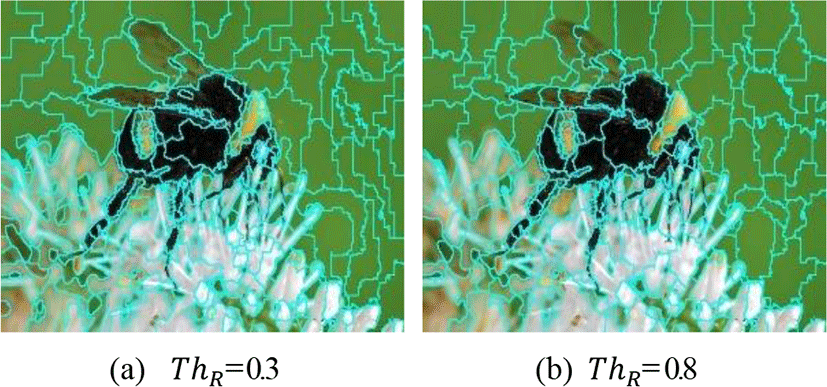Fig. 5. Superpixel images with roundness thresholds.

Fig. 6 shows the results of magnifying the head area for precise analysis. Fig. 6(a) and Fig. 6(b) are the head parts of Fig. 3 and Fig. 5(b), respectively. It can be seen that the proposed method allocates more superpixels to a complex area than the SLIC method, and preserves the boundaries of the objects more accurately.Fig. 6. Enlarged images of the bee’s head area.

We analyzed the performance of the proposed method for various images. Figure 7 shows the results of applying the superpixel algorithms to four images from the Berkeley database . The target number of superpixels is set to 300, and parameter α is set to 5. The first row shows the original images, and the second row shows the results of applying the SLIC method. The third and fourth rows show the results of applying the proposed method with parameter ThR values of 0.3 and 0.8, respectively. The images in the fifth and sixth rows are the results of magnifying the areas containing complex elements in the images of the second and fourth rows, respectively. The third and fourth rows show that the proposed method can adequately control the shapes of superpixels. It can be seen from the images in the fifth row and sixth row that the proposed method segments the region more accurately than the SLIC method by allocating many superpixels to the intricate areas of the images.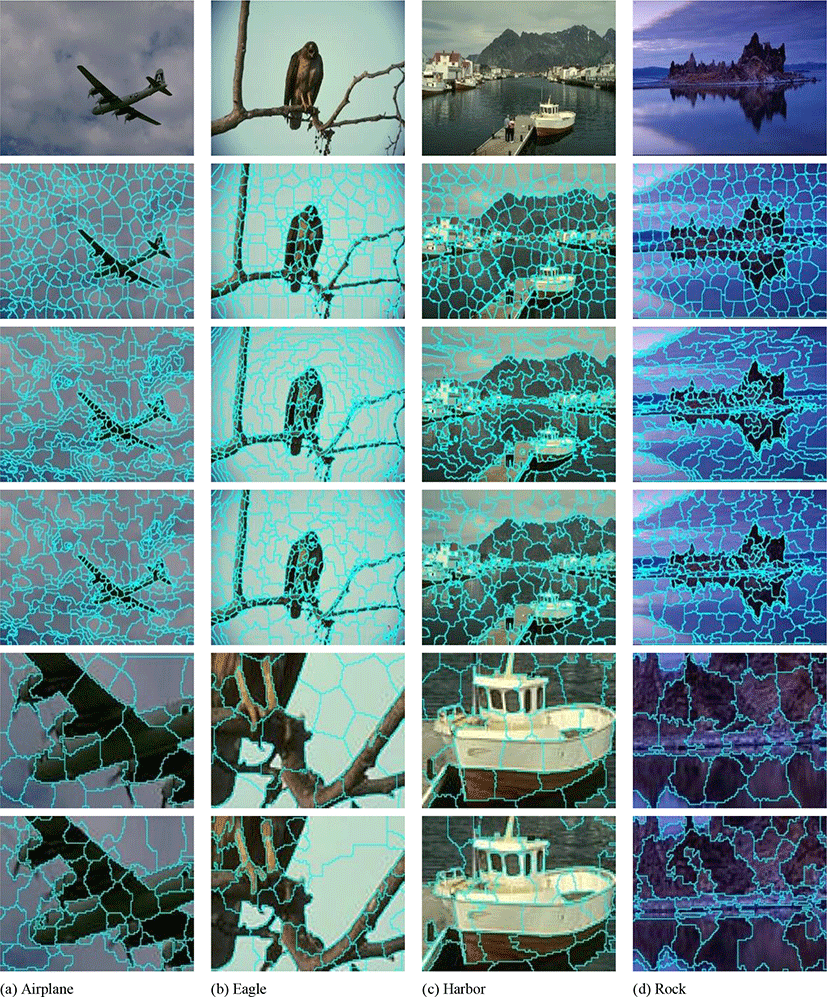Fig. 7. Performance comparison with four images.

It is examined how accurately the superpixels are generated for the target number of superpixels. Table 1 shows the target number of superpixels and the number of actually generated superpixels for five images. Since the SLIC method does not guarantee perfect connectivity for all pixels, it can be seen that the number of generated superpixels is less than the target number . Since the proposed method continues merging process until the number of superpixels reaches the target number, the number of generated superpixels matches the target number.

Table 1. Comparison of the number of generated superpixels.
Image N* Number of superpixels
SLIC Proposed
Bee 300 279 300
Airplane 200 267 300
Eagle 300 265 300
Harbor 300 275 300
Rock 300 256 300

The execution speed of the proposed method was compared with that of the SLIC method. The implementations were run on a 3.50GHz processor with 16GB of RAM. Table 2 shows the execution time of the proposed method and the SLIC method for five images. It can be seen that the proposed method takes about two to three times the execution speed of the SLIC method. To analyze the processing time of the proposed method, the proposed method was divided into the over-segmentation step, the graph generation step, and the superpixel merging step. Table 2 shows the step-by-step processing time of the proposed method. The proposed method takes more time than the SLIC method because the SLIC method is used in the over-segmentation step. It can be seen that it takes a lot of time to generate a graph for the over-segmented superpixels compared with the time to merge using the graph. It is because it takes a long time to create the feature vectors of all the superpixels and create an ordered graph of them.

Table 2. Comparison of the execution speed.
Image SLIC (sec) Proposed (sec)
SLIC Graph generation Superpixel Merging Sum
Bee 0.108 0.119 0.109 0.029 0.257
Airplane 0.065 0.068 0.091 0.024 0.183
Eagle 0.065 0.070 0.085 0.021 0.176
Harbor 0.067 0.072 0.096 0.023 0.191
Rock 0.053 0.072 0.093 0.023 0.188

The most important property of a superpixel method is its ability to adhere to object boundaries, and boundary recall is standard measure for boundary adherence . Boundary recall measures what fraction of the ground truth edges fall within at least two pixels of a superpixel boundary. Fig. 8 shows the result of measuring boundary recall for 300 superpixels generated by applying the proposed method to the eagle image. The recall value of the superpixels created using the SLIC method is 0.86. It can be seen that the boundary recall of the proposed method is superior to the SLIC method. Fig. 8 shows that increasing the maximum size of superpixels improves the boundary recall performance. In addition, it can be seen that reducing the roundness threshold value improves the boundary recall performance. However, as the maximum size of the superpixels increases and the roundness threshold value decreases, shapes of the superpixels become irregular. Therefore, when applying the proposed method to an application, it is necessary to set the parameters appropriately for the purpose.Fig. 8. Segmentation performance comparison in terms of boundary recall.

## V. CONCLUSION

In this paper, we proposed a superpixel generation method that can adequately adjust the shapes of superpixels. Since superpixel segmentation is used in the preprocessing stage in computer vision applications, it is common to create superpixels in similar shapes and sizes. However, in order to accurately segment the boundaries of the objects in an image, it is advantageous to create superpixels with various shapes by reflecting the contents of the image. In this paper, we propose a method of generating superpixels with various shapes. The proposed method consists of two steps. The first step focuses on preserving the boundaries of the objects in an image and creates a sufficient number of superpixels. In the second step, superpixels are merged by reflecting the complexity of the area in the image. The shapes of superpixels are controlled by limiting the size and roundness. The experimental results confirmed that the proposed method maintains the boundaries of objects well by generating superpixels of various shapes in consideration of the complexity of the area. In this paper, the focus was not on processing speed. Thus, the proposed method takes about two to three times the execution speed of the SLIC method. In the future study, we will cover how to save time when generating graphs.

## REFERENCES

.

J. Kim, “The Object Image Detection Method using statistical properties,” Journal of the Korea Institute of Information and Communication Engineering, vol. 22. no. 7, pp. 956-962, Jul. 2018..

J. Yao, M. Boben, S. Fidler, and R. Urtasun, “Real-time coarse-to-fine topologically preserving segmentation,” in Proceedings of the IEEE Conference on Computer Vision and Pattern Recognition, Boston, pp. 2947-2955, 2015..

R. Giraud, V. T. Ta, and N. Papadakis. “Evaluation framework of superpixel methods with a global regularity measure,” Journal of Electronic Imaging, vol. 26, no. 6, pp. 1-18, 2017..

D. Comaniciu and P. Meer, “Mean shift: A robust approach toward feature space analysis,” IEEE Transactions on Pattern Analysis and Machine Intelligence, vol. 24, no. 5, pp. 603-619, May 2002..

P. Felzenszwalb and D. Huttenlocher, “Efficient graph-based image segmentation,” International Journal of Computer Vision, vol. 59, no. 2, pp.167-181, Sep. 2004..

A. Levinshtein, A. Stere, K. Kutulakos, D. Fleet, S. Dickinson, and K. Siddiqi, “Turbopixels: fast superpixels using geometric flows,” IEEE Transactions on Pattern Analysis and Machine Intelligence, vol. 31, no. 12, pp. 2290-2297, Dec. 2009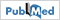.

D. Stutz, A. Hermans, and B. Leibe. “Superpixels: An evaluation of the state-of-the-art,” Computer Vision and Image Understanding, vol. 166, pp. 1-27, Jan. 2018..

A. Rubio, L. Yu, E. Simo-Serra, and F. Moreno-Noguer, “BASS: boundary-aware superpixel segmentation,” In Proceeding of the International Conference on Pattern Recognition, Cancun, pp. 2824-2829, 2016..

M. Reso, J. Jachalsky, B. Rosenhahn, and J. Ostermann, “Temporally consistent superpixels," in Proceedings of the IEEE International Conference on Computer Vision, Sydney, pp. 385-392, 2013..

S. Mukherjee, R. Saini, P. Kumar, P. P. Roy, D. P. Dogra, and B. G. Kim, “Fight Detection in Hockey Videos using Deep Network,” Journal of Multimedia Information System, vol. 4, no. 4, pp. 225-232, 2017..

C. Wang, Z. Liu, and S. C. Chan, “Superpixel-based Hand Gesture Recognition with Kinect Depth Camera,” IEEE Transactions on Multimedia, vol. 17, no. 1, pp. 29-39, 2014..

A. Mittal, P. P. Roy, P. Singh, and B. Raman, “Rotation and script independent text detection from video frames using sub pixel mapping,” Journal of Visual Communication and Image Representation, vol. 46, pp. 187-198, 2017..

S. Gupta, P. P. Roy, D. P. Dogra, and B. G. Kim, “Retrieval of colour and texture images using local directional peak valley binary pattern,” Pattern Analysis and Applications, vol. 23, pp. 1-17, 2020..

J. H. Kim, B. G. Kim, P. P. Roy, and D. M. Jeong, “Efficient Facial Expression Recognition Algorithm Based on Hierarchical Deep Neural Network Structure,” IEEE Access, vol. 7, pp. 41273-41285, 2019..

M. Soltaninejad, G. Yang, T. Lambrou, N. Allinson, T. L. Jones, T. R. Barrick, F. A. Howe, and X. Ye, “Automated brain tumour detection and segmentation using superpixel-based extremely randomized trees in FLAIR MRI,” International Journal of Computer Assisted Radiology and Surgery, vol. 12, no. 2, pp. 183-203, 2017..

W. Qin, J. Wu, F. Han, Y. Yuan, W. Zhao, B. Ibragimov, J. Gu, and L. Xing, “Superpixel-based and boundary-sensitive convolutional neural network for automated liver segmentation,” Physics in Medicine & Biology, vol 63. no. 9. pp. 1-14, 2018..

R. Achanta, A. Shaji, K. Smith, A. Lucchi, P. Fua, and S. Süsstrunk, “Slic superpixels compared to state-of-the-art superpixel methods,” IEEE Transaction on Pattern Analysis and Machine Intelligence, vol. 34, no. 11, pp. 2274–2282, Nov. 2012..

D. Martin, C. Fowlkes, D. Tal, and J. Malik, “A database of human segmented natural images and its application to evaluating segmentation algorithm and measuring ecological statistics”, in Proceedings of IEEE International Conference Computer Vision, Vancouver, pp. 416-423, 2001.## Author

Sanghyun Park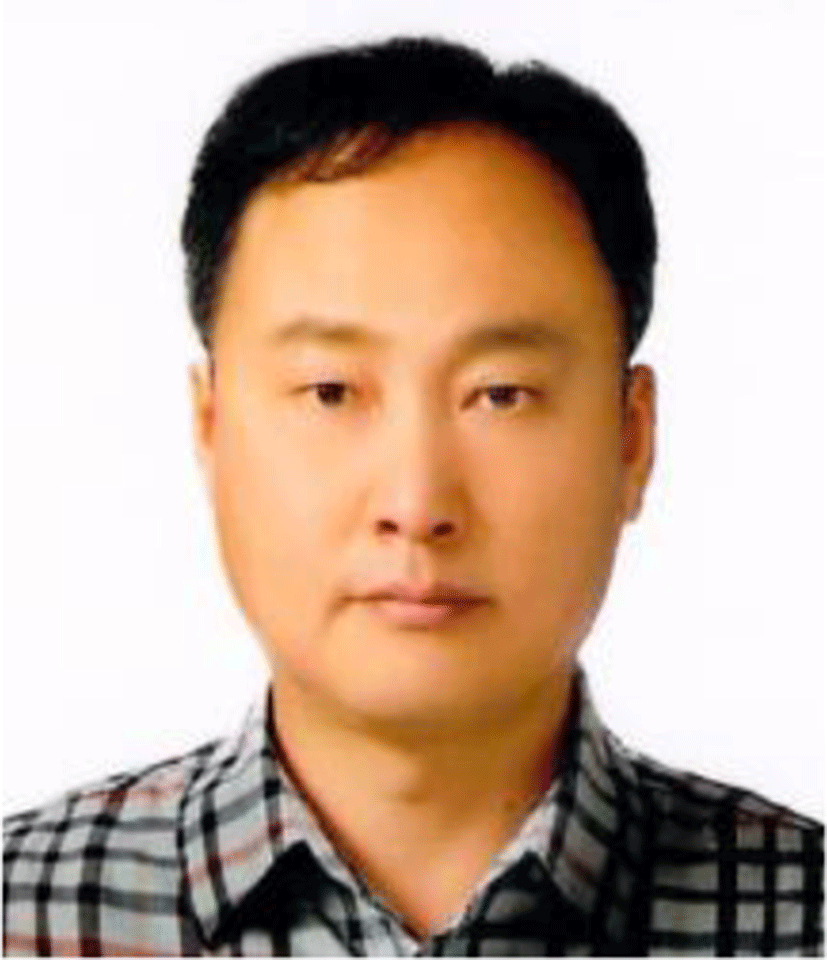received his B.S., M.S., and Ph.D. degrees from Korea University, Seoul Korea in 1995, 1997, and 2002, respectively. He is now a professor in Department of Multimedia Engineering, Sunchon National University, Korea.

His research interests include image processing, computer vision, and multimedia system.